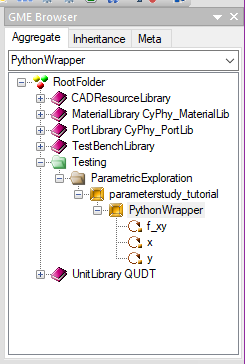# Adding a PET Analysis Block¶

At this point in the tutorial, we have created a PET (parameterstudy_tutorial) in GME; however, our PET is empty (and useless). Let’s add a model that we can analyze to parameterstudy_tutorial.

1. Locate the PythonWrapper within the Part Browser window (you may have to scroll down to find it).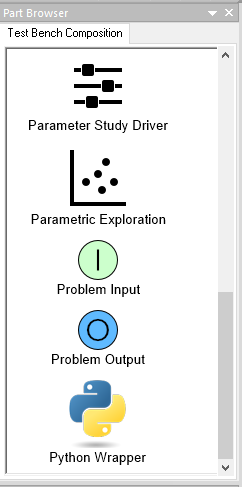1. Left-click on the PythonWrapper icon and drag it onto the PET canvas.The PythonWrapper Component allows Python scripts that are formatted to run within the underlying OpenMDAO framework to be exposed as a block within OpenMETA. We are going to model our Paraboloid using a Python script and then load that script into the PythonWrapper Component in our PET.

Note

For more information on OpenMDAO Component scripts and how to write them, reference the OpenMDAO documentation: http://openmdao.readthedocs.io/en/1.7.3/usr-guide/basics.html#component

1. Open Notepad (or your favorite text editor) and copy-paste in the code below.
```from __future__ import print_function
from openmdao.api import Component
from pprint import pprint

''' First, let's create the component defining our system. We'll call it 'Paraboloid'. '''
class Paraboloid(Component):
''' Evaluates the equation f(x,y) = (x-3)^2 +xy +(y+4)^2 - 3 '''

def __init__(self):
super(Paraboloid, self).__init__()

''' Inputs to the PythonWrapper Component are added here as params '''

''' Outputs from the PythonWrapper Component are added here as unknowns '''

def solve_nonlinear(self, params, unknowns, resids):
''' This is where we describe the system that we want to add to OpenMETA '''
''' f(x,y) = (x-3)^2 + xy + (y+4)^2 - 3 '''

x = params['x']
y = params['y']

f_xy = (x-3.0)**2 + x*y + (y+4.0)**2 - 3.0

unknowns['f_xy'] = f_xy

''' This is an equivalent expression to the one above
unknowns['f_xy'] = (params['x']-3.0)**2 + params['x']*params['y'] + (params['y']+4.0)**2 - 3.0
'''
```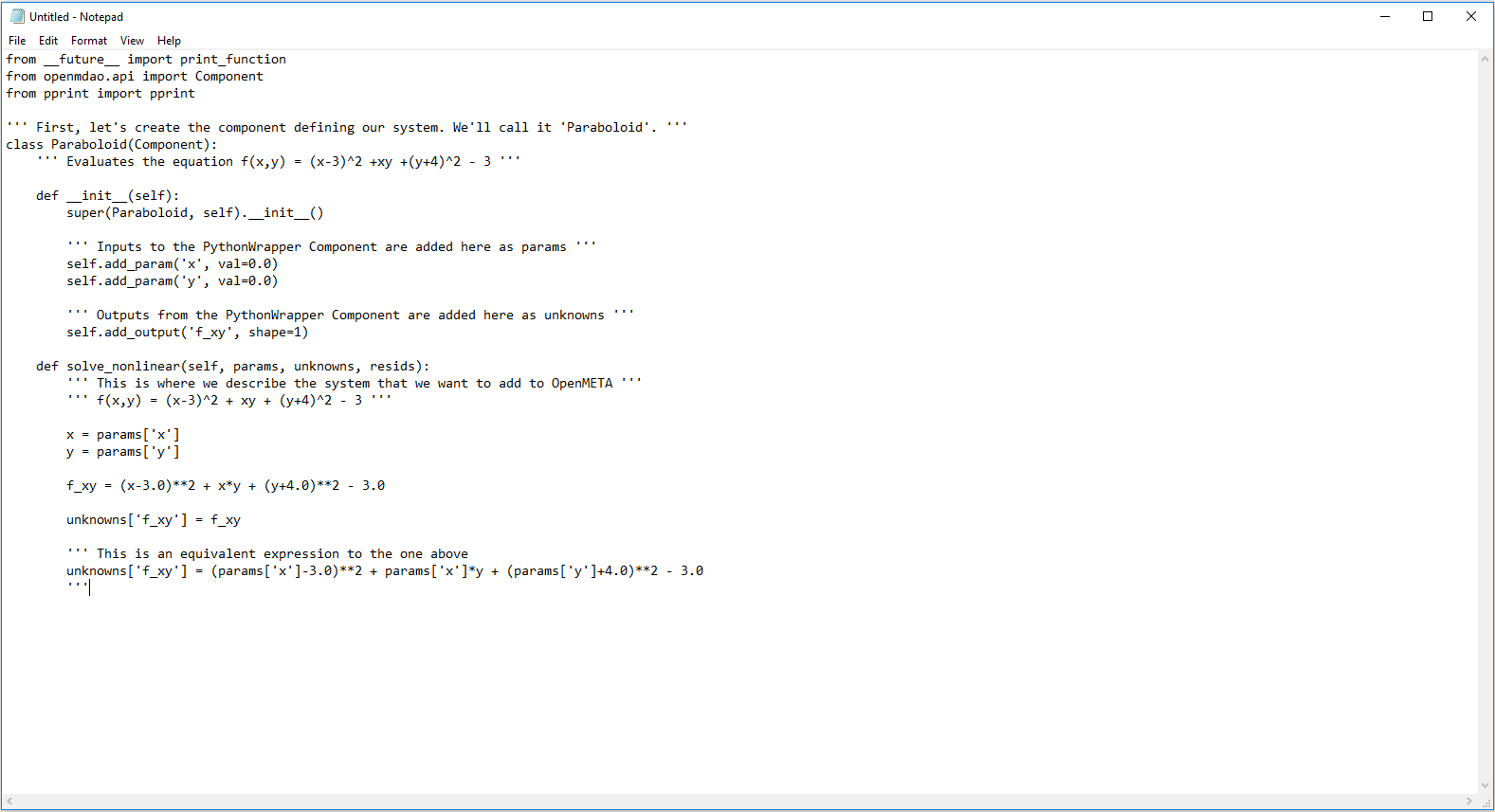1. Select File ‣ Save As…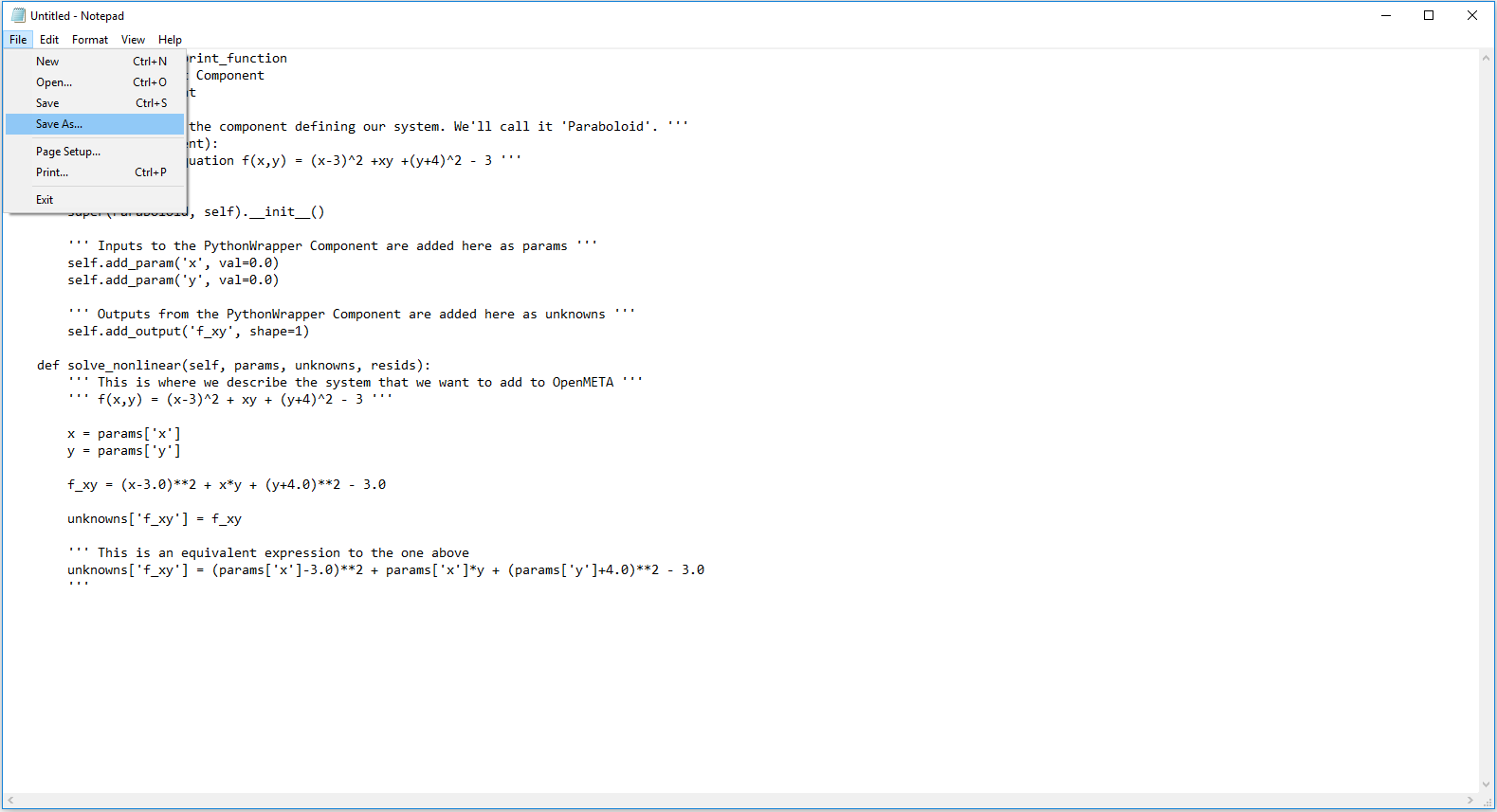5. A Save As window will appear. Make a new folder named scripts within your GME project folder.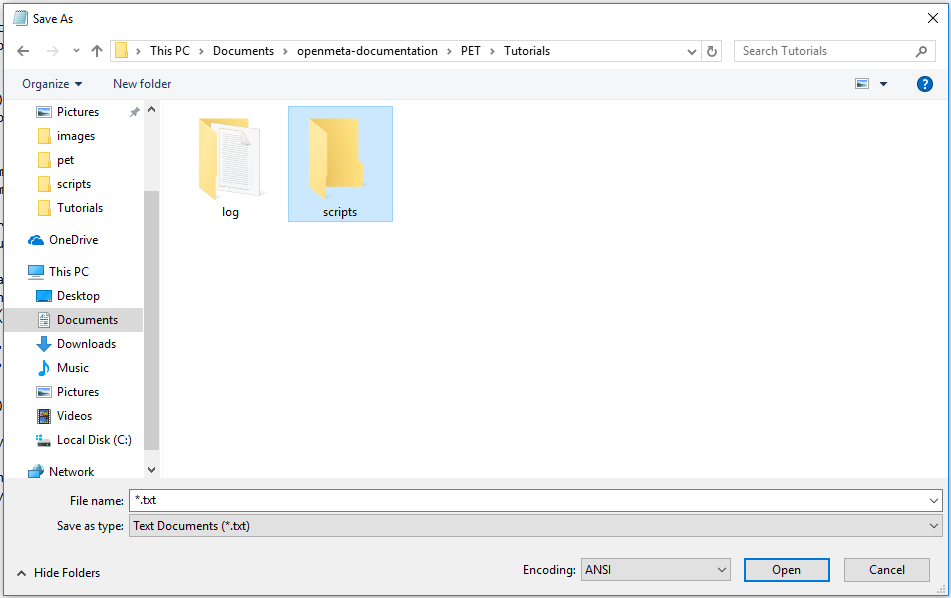1. Save the file as “paraboloid.py” within the scripts folder.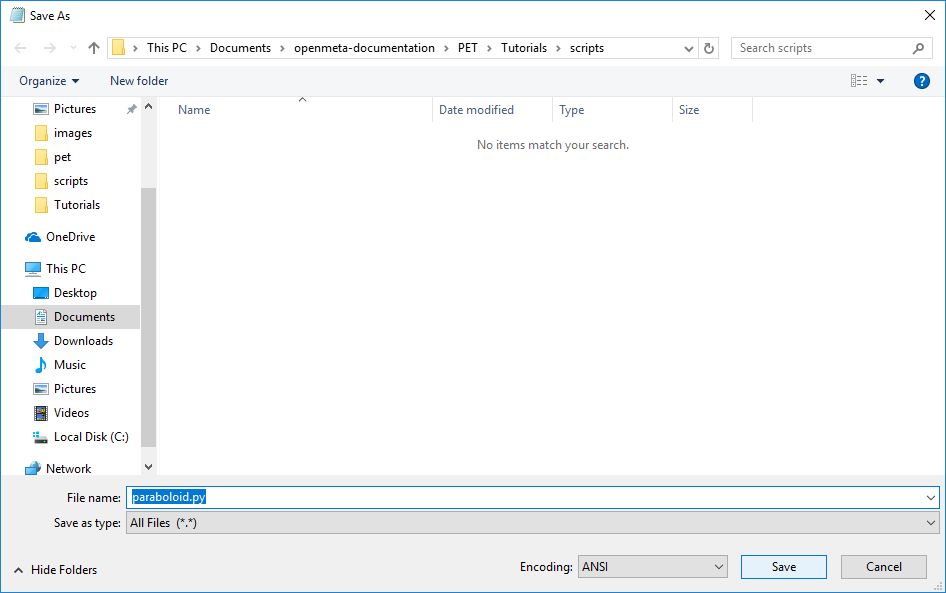Note

If you are using Notepad, make sure Save as type is set to `All Files (*.*)`.

2. Double-click on the PythonWrapper Component in the PET canvas.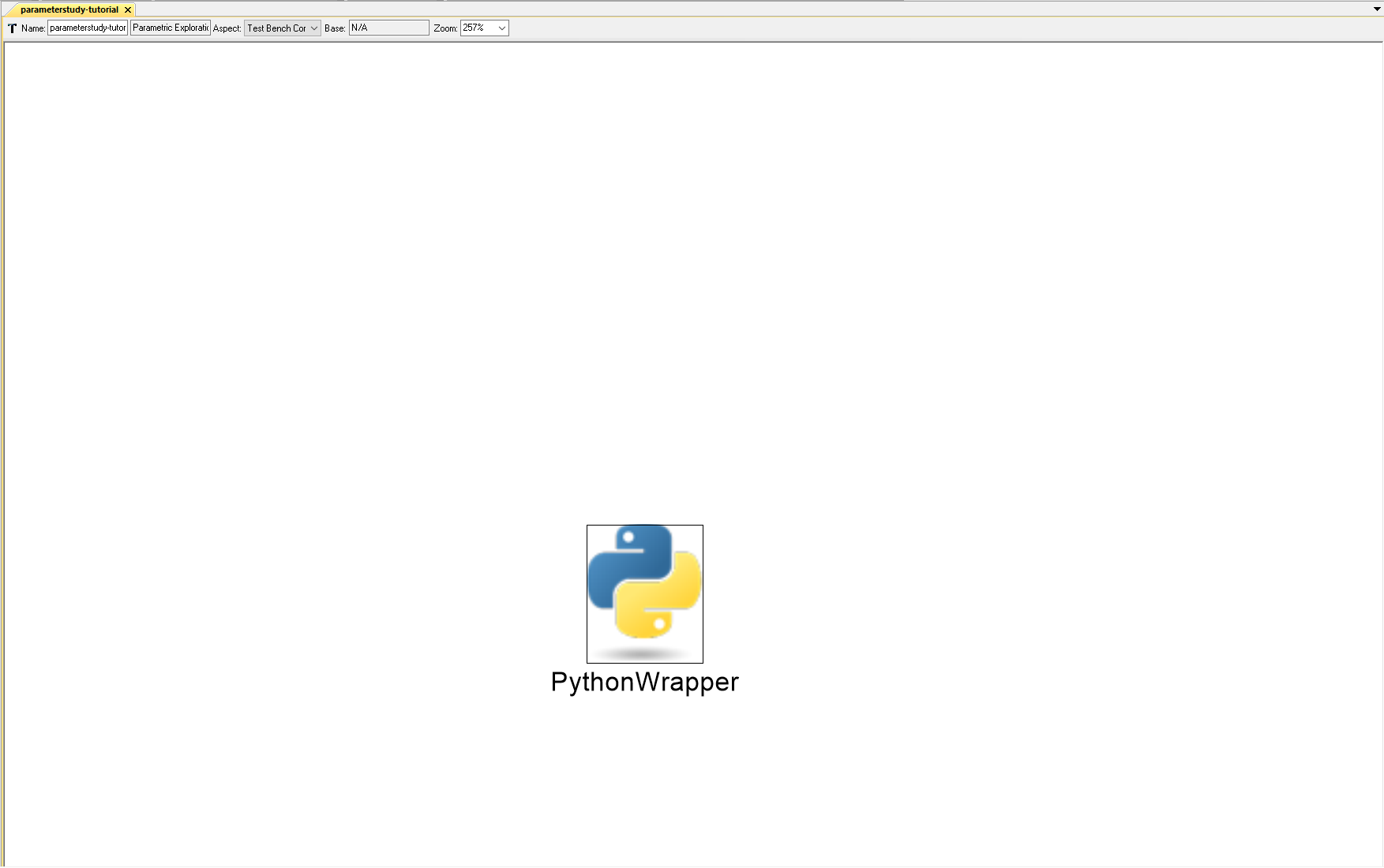A file explorer will open.

1. Navigate to the location of paraboloid.py.
2. Select paraboloid.py and click Open.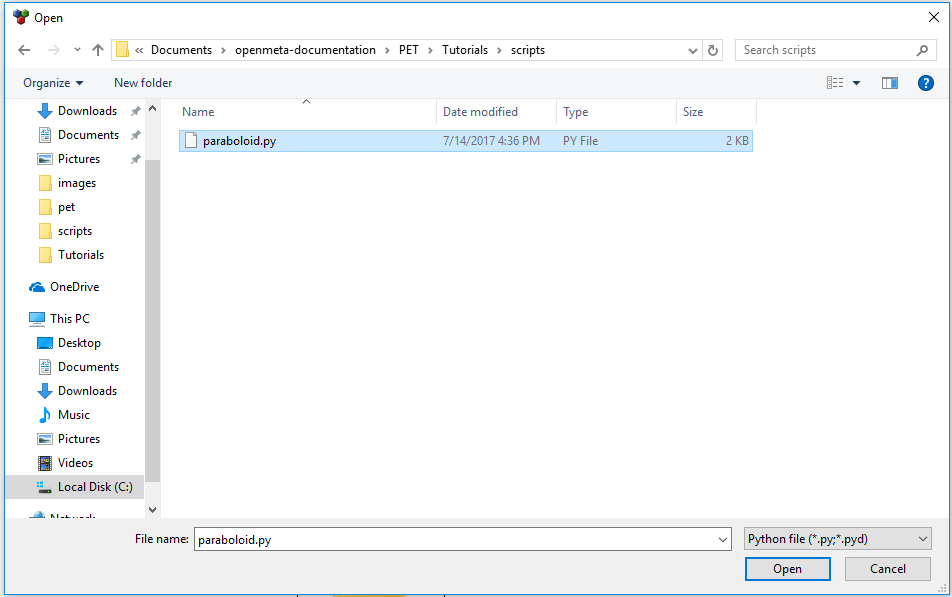The PythonWrapper Component model will change. Notice that the params and unknowns from the paraboloid.py script are now exposed as ports on the outside of the PythonWrapper Component model.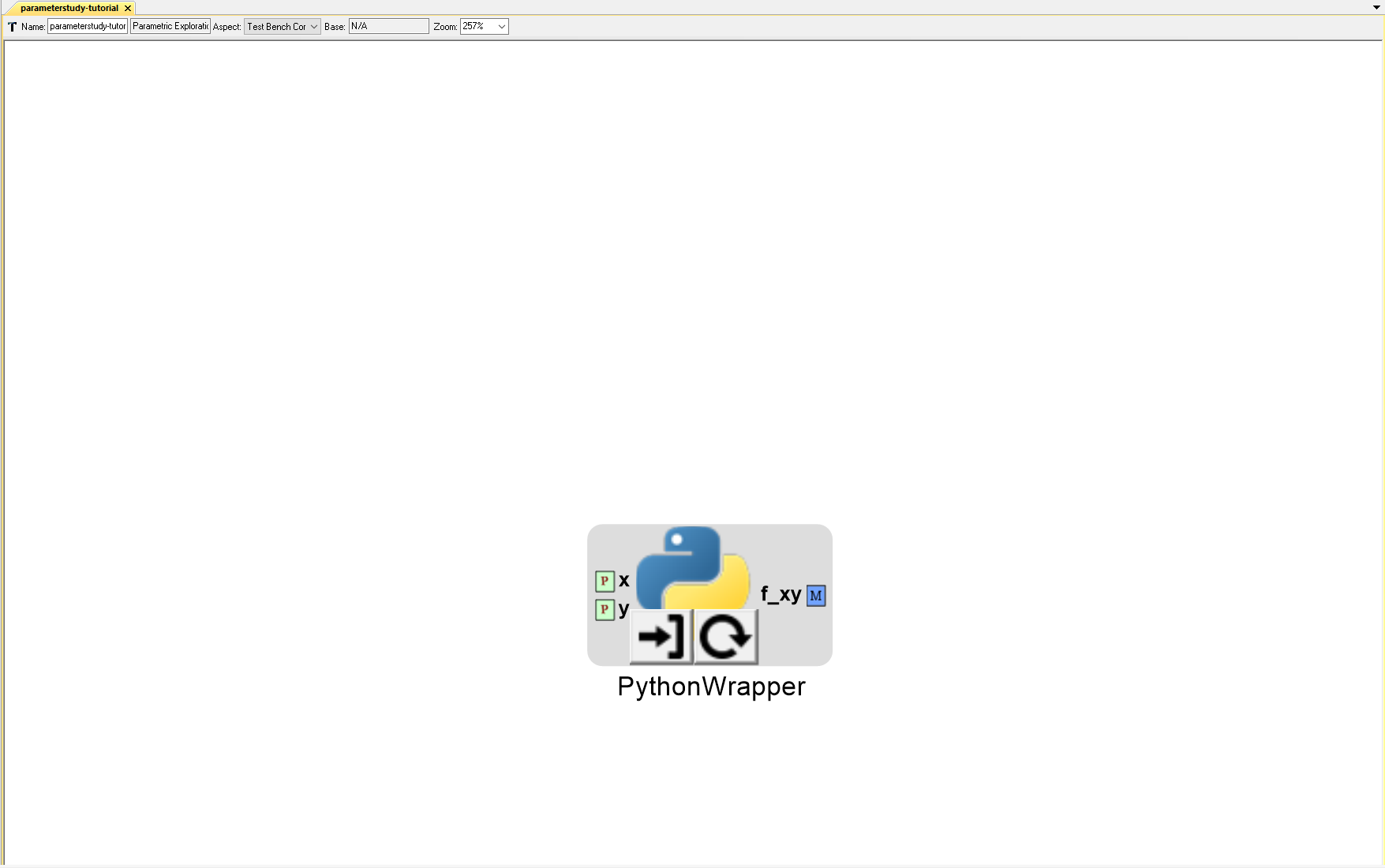Also, remember that the PET’s hierarchy can be viewed in the GME Browser window.